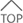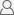会员登录 | 一账通登录 | 免费注册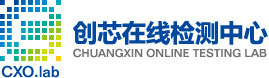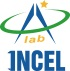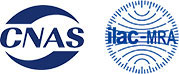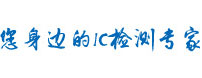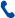4008-655-800重量单位换算

• 公斤(kg)

• 克(g)

• 毫克(mg)

• 市斤

• 金衡磅(lb t)

• 金衡盎司(oz t)

• 英钱(dwt)

• 金衡格令

### 【常 衡 制】

• (英制)长吨

• (美制)短吨

• 英担(cwt)

• 美担(cwt)

• 英石

• 磅(lb)

• 盎司(oz)

• 打兰(dr)

• 格令

1 吨= 1,000,000 克

1 公斤 = 1,000 克

1 毫克 = 0.001 克

1 微克 = 0.000 001克

1 担=50 kg

1 斤=0.5 kg

1 两=0.05 kg

1 钱=0.005 kg

1 grain 格令 =0.065 gram 克

1 dram 打兰 =1.772 grams 克

1 ounce 盎司 =16 drams 打兰 =28.35 grams 克

1 pound 磅 =16 ounces 盎司 =7000 grains 谷 =0.4536 kilogram 千克

1 stone 英石 =14 pounds 磅 =6.35 kilograms 千克

1 quarter 四分之一英担 =2 stones 英石 =12.70 kilograms 千克

1 hundredweight 英担 =4 quarters 四分之一英担 =50.80 kilograms 千克

1 short ton 短吨 ( 美吨 )=2000 pounds 磅 =0.907 tonne 公吨

1 (long) ton 长吨 ( 英吨 )=20 hundredweight 英担 =1.016 tonnes 公吨相关推荐
•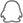QQ咨询

•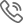咨询热线

咨询热线：

0755-82719442
0755-23483975

•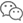官方微信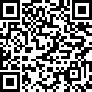欢迎关注官方微信

CXOLab创芯在线
检测实验室

•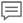意见反馈

•